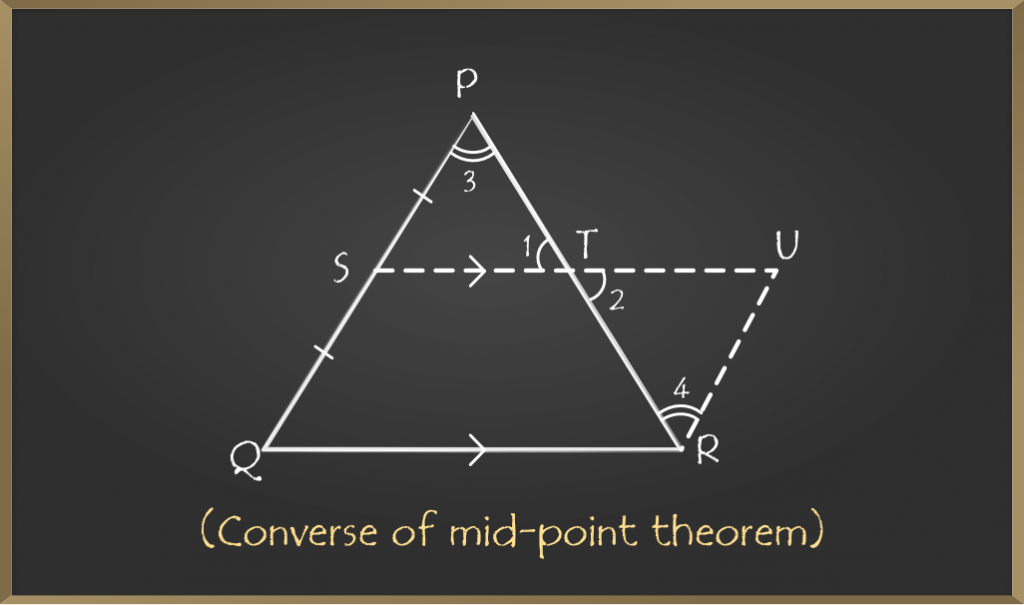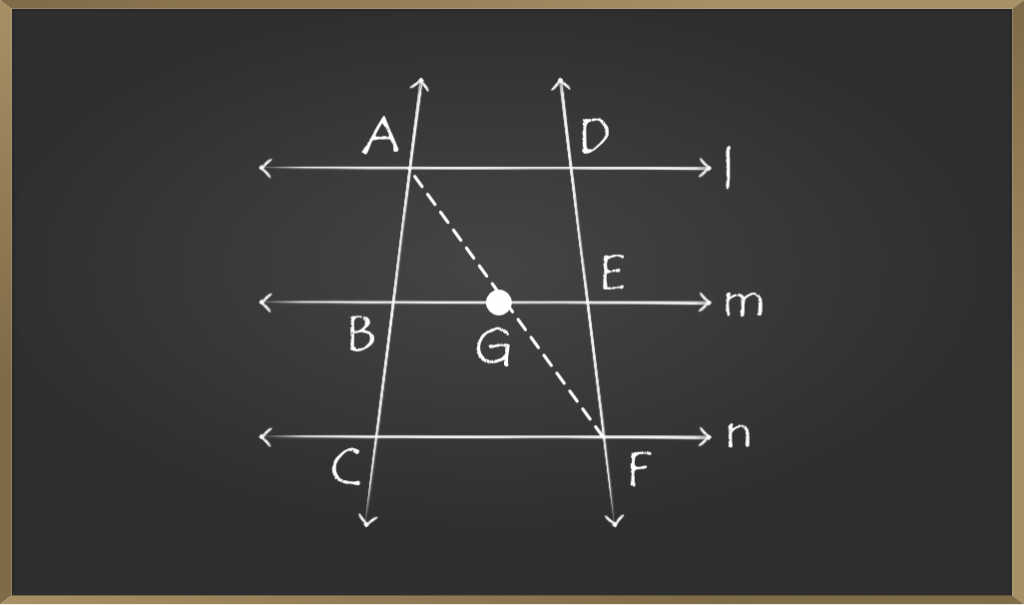GFG App
Open AppBrowser
Continue

The midpoint theorem states that “The line segment drawn from the midpoint of two sides of the triangle is parallel to the third side and is equal to half of the third side of the triangle. Geometry is an important part of mathematics that deals with different shapes and figures. Triangles are an important part of geometry and the mid-point theorem points towards the midpoints of the triangle.

## Midpoint Theorem Definition

The midpoint theorem states that the line segment joining the midpoints of any two sides of a triangle is parallel to the third side and equal to half of the third side. Consider a triangle, ΔABC. Let D and E be the midpoints of AB and AC respectively, now join DE. Then the midpoint theorem states that DE is parallel to BC and equal to half of BC.

## Mid-Point Theorem Proof

This theorem states that” The line segment joining mid-points of two sides of a triangle is parallel to the third side of the triangle and is half of it”Given:

A triangle ABC in which D is the mid-point of AB and E is the mid-point of AC.

To Prove:

DE ∥ BC and DE = 1/2(BC)

Construction:

Extend the line segment joining points D and E to F such that DE = EF and join CF.

Proof:

In ∆AED and ∆CEF

DE = EF (construction)
∠1 = ∠2 (vertically opposite angles)
AE = CE (E is the mid-point)

△AED ≅ △CEF by SAS criteria

Therefore,

∠3 =∠4   (c.p.c.t)

But these are alternate interior angles.

So, AB ∥ CF

But AD = DB (D is the mid-point)

Therefore, BD = CF

In BCFD

BD∥ CF (as AB ∥ CF)

BD = CF

BCFD is a parallelogram as one pair of opposite sides is parallel and equal.

Therefore,

DF∥ BC (opposite sides of parallelogram)

DF = BC (opposite sides of parallelogram)

As DF∥ BC, DE∥ BC and DF = BC

But DE = EF

So, DF = 2(DE)

2(DE) = BC

DE = 1/2(BC)

Hence, proved that the line joining the mid-points of two sides of the triangle is parallel to the third side and is half of it.

## Converse of Mid-Point Theorem

The line drawn through the mid-point of one side of a triangle parallel to the base of a triangle bisects the third side of the triangle.Given:

In triangle PQR, S is the mid-point of PQ and ST ∥ QR

To Prove:

T is the mid-point of PR.

Construction:

Draw a line through R parallel to PQ and extend ST to U.

Proof:

ST∥ QR   (given)

So, SU∥ QR

PQ∥ RU   (construction)

Therefore, SURQ is a parallelogram.

SQ = RU   (Opposite sides of parallelogram)

But SQ = PS   (S is the mid-point of PQ)

Therefore, RU = PS

In △PST and △RUT

∠1 =∠2   (vertically opposite angles)
∠3 =∠4   (alternate angles)
PS = RU   (proved above)

△PST ≅ △RUT by AAS criteria

Therefore, PT = RT

T is the mid-point of PR.

## MidPoint Formula

Midpoint of any line segment is defined as the coordinate point that divides the line segment into two equal parts.

Suppose P(x1, y1) and Q(x2, y2) are the coordinates of endpoints of any line segment, then the midpoint formula is given as:

Midpoint = [(x1 + x2)/2, (y1 + y2)/2]

## Solved Examples on Mid Point Theorem

Example 1: l, m, and n are three parallel lines. p and q are two transversals intersecting parallel lines at A, B, C, D, E, and F as shown in the figure. If AB:BC = 1:1, find the ratio of DE:EF.Given: AB:BC = 1:1

To find: DE:EF

Construction: Join AF such that it intersects line m at G.

In △ACF

AB = BC (1:1 ratio)

BG∥ CF (as m∥n)

Therefore, by converse of mid-point theorem G is the midpoint of AF (AG = GF)

Now, in △AFD

AG = GF (proved above)

Therefore, by converse of mid-point theorem E is the mid-point of DF (FE = DE)

So, DE:EF = 1:1 (as they are equal)

Example 2: In the figure given below L, M and N are mid-points of side PQ, QR, and PR respectively of triangle PQR.

If PQ = 8cm, QR = 9cm and PR = 6cm. Find the perimeter of the triangle formed by joining L, M, and N.Solution:

As L and N are mid-points

By mid-point theorem

LN ∥ QR and LN = 1/2 × (QR)

LN = 1/2 × 9 = 4.5cm

Similarly, LM = 1/2 × (PR) = 1/2×(6) = 3cm

Similarly, MN = 1/2 × (PQ) = 1/2 × (8) = 4cm

Therefore, the perimeter of △LMN = LM + MN + LN

= 3 + 4 + 4.5

= 11.5 cm

Perimeter is 11.5cm

## FAQs on MidPoint Theorem

Question 1: What is meant by the midpoint theorem?

Midpoint theorem states that “The line joining the midpoint of two sides of the triangle is parallel to its third side of the triangle and is also half of it.”

Question 2: What is the converse of the midpoint theorem?

Converse of mid point theorem states that,” The line drawn through the midpoint of any one side of a triangle, and parallel to another side bisects the third side.

Question 3: What is the definition of a midpoint?